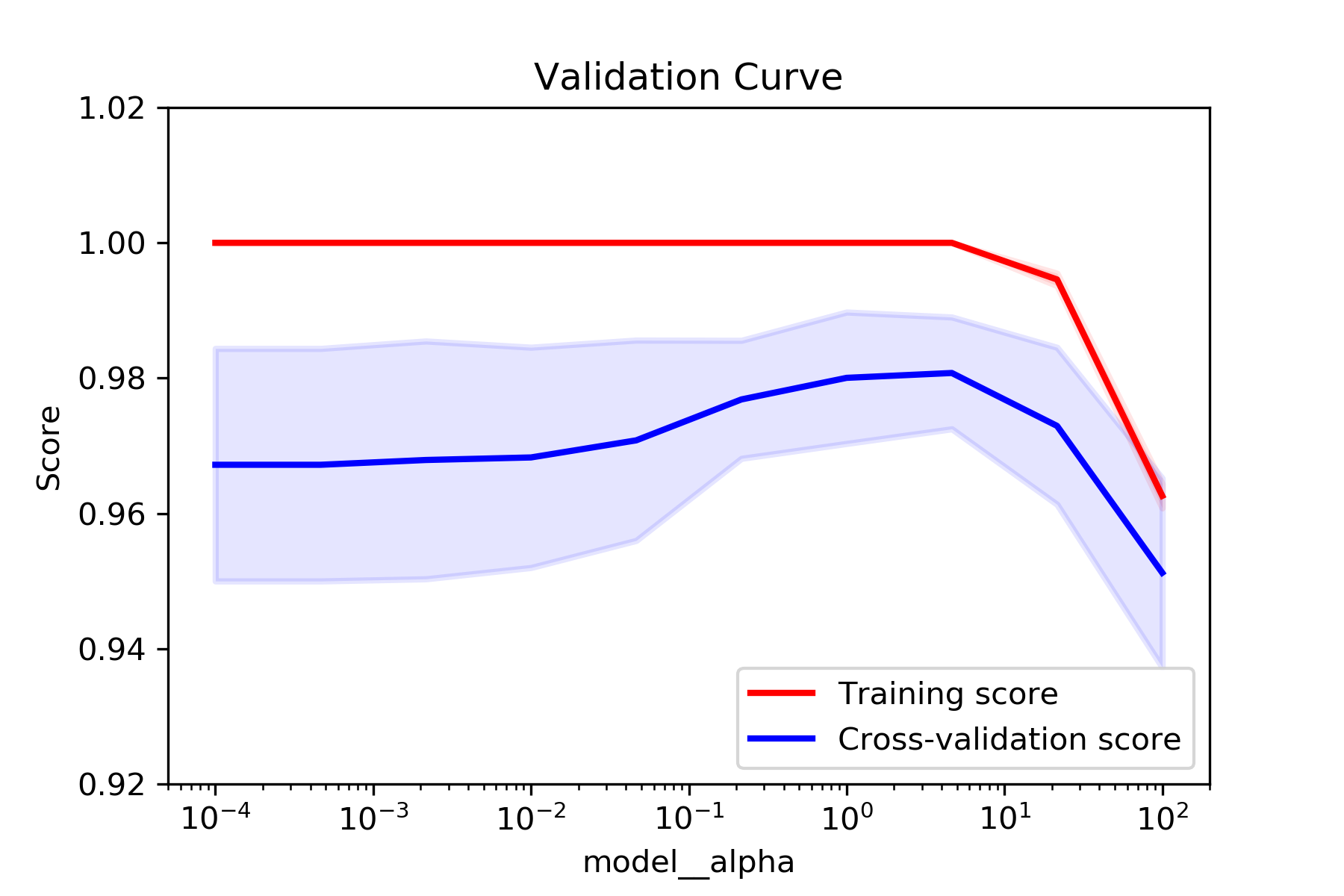# Validation Curve Plot from GridSearchCV Results

For a course in machine learning I’ve been using sklearn’s GridSearchCV to find the best hyperparameters for some supervised learning models. I wanted to fix all but one of the hyperparameters to be set to the `best_params_` values, and then plot the model’s performance as a single parameter was varied. It can be time consuming to run those experiments directly with `modelselection.validation_curve()`, and the search grid already contains scores for various parameter values. I found examples of plotting the grid’s `cv_results_` when a couple of parameters are considered, but some of my grid searches were over more parameters that I wanted to plot.

So I wrote this function which will plot the training and cross-validation scores from a `GridSearchCV` instance’s results:

``````def plot_grid_search_validation_curve(grid, param_to_vary,
title='Validation Curve', ylim=None,
xlim=None, log=None):
"""Plots train and cross-validation scores from a GridSearchCV instance's
best params while varying one of those params."""

df_cv_results = pd.DataFrame(grid.cv_results_)
train_scores_mean = df_cv_results['mean_train_score']
valid_scores_mean = df_cv_results['mean_test_score']
train_scores_std = df_cv_results['std_train_score']
valid_scores_std = df_cv_results['std_test_score']

param_cols = [c for c in df_cv_results.columns if c[:6] == 'param_']
param_ranges = [grid.param_grid[p[6:]] for p in param_cols]
param_ranges_lengths = [len(pr) for pr in param_ranges]

train_scores_mean = np.array(train_scores_mean).reshape(*param_ranges_lengths)
valid_scores_mean = np.array(valid_scores_mean).reshape(*param_ranges_lengths)
train_scores_std = np.array(train_scores_std).reshape(*param_ranges_lengths)
valid_scores_std = np.array(valid_scores_std).reshape(*param_ranges_lengths)

param_to_vary_idx = param_cols.index('param_{}'.format(param_to_vary))

slices = []
for idx, param in enumerate(grid.best_params_):
if (idx == param_to_vary_idx):
slices.append(slice(None))
continue
best_param_val = grid.best_params_[param]
idx_of_best_param = 0
if isinstance(param_ranges[idx], np.ndarray):
idx_of_best_param = param_ranges[idx].tolist().index(best_param_val)
else:
idx_of_best_param = param_ranges[idx].index(best_param_val)
slices.append(idx_of_best_param)

train_scores_mean = train_scores_mean[tuple(slices)]
valid_scores_mean = valid_scores_mean[tuple(slices)]
train_scores_std = train_scores_std[tuple(slices)]
valid_scores_std = valid_scores_std[tuple(slices)]

plt.clf()

plt.title(title)
plt.xlabel(param_to_vary)
plt.ylabel('Score')

if (ylim is None):
plt.ylim(0.0, 1.1)
else:
plt.ylim(*ylim)

if (not (xlim is None)):
plt.xlim(*xlim)

lw = 2

plot_fn = plt.plot
if log:
plot_fn = plt.semilogx

param_range = param_ranges[param_to_vary_idx]
if (not isinstance(param_range, numbers.Number)):
param_range = [str(x) for x in param_range]
plot_fn(param_range, train_scores_mean, label='Training score', color='r',
lw=lw)
plt.fill_between(param_range, train_scores_mean - train_scores_std,
train_scores_mean + train_scores_std, alpha=0.1,
color='r', lw=lw)
plot_fn(param_range, valid_scores_mean, label='Cross-validation score',
color='b', lw=lw)
plt.fill_between(param_range, valid_scores_mean - valid_scores_std,
valid_scores_mean + valid_scores_std, alpha=0.1,
color='b', lw=lw)

plt.legend(loc='lower right')

plt.show()
``````
``````plot_grid_search_validation_curve(grid__digits, 'model__alpha', log=True, ylim=(.92, 1.02))
``````